# lookup方法内只允许使用子查询（let+pipeline）方式

const db = uniCloud.database()
let res = await db.collection('orders').aggregate()
.lookup({
from: 'books',
localField: 'book',
foreignField: 'title',
as: 'bookList',
})
.end()

2021-04-22 00:01 分享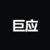DaZiYuan (作者) - 巨应工作室首席打字员

chunk-vendors.js:2261 Uncaught (in promise) Error: lookup方法内只允许使用子查询（let+pipeline）方式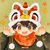``````const dbCmd = db.command
const \$ = dbCmd.aggregate
db.collection('epidemics')
.aggregate().lookup({
from: '表名',
let: {
某某_id: '\$某某_id'
},
pipeline: \$.pipeline()
.match(dbCmd.expr(
\$.eq(['\$_id', '\$\$某某_id'])
))
.done(),
as: '某某'
}).lookup({
from: '表名1',
let: {
某某1_id: '\$某某1_id'
},
pipeline: \$.pipeline()
.match(dbCmd.expr(
\$.eq(['\$_id', '\$\$某某1_id'])
))
.done(),
as: '某某1'
}).end().then((res) => {
console.log(res.result.data)

}).catch(error => console.log(error))``````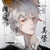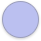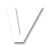const db = uniCloud.database()
const dbCmd = db.command
await db.collection('表单1')
.aggregate()
.match({
"_id": dbCmd.in(ides)
})
.lookup({
from: '表单2',
localField: '_id',
foreignField: '外键',
as: '重命名'
})
.lookup({
from: '表单3',
localField: '_id',
foreignField: '外键',
as: '重命名'
})
.end();